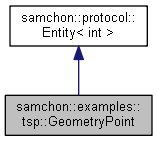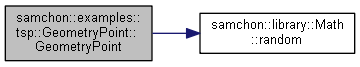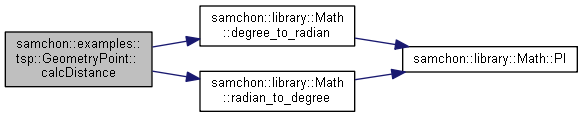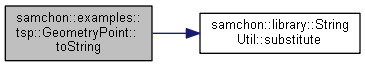Samchon Framework for CPP  1.0.0
samchon::examples::tsp::GeometryPoint Class Reference

A geometry coordinates (point) More...

`#include <GeometryPoint.hpp>`

Collaboration diagram for samchon::examples::tsp::GeometryPoint:[legend]

## Public Member Functions

GeometryPoint ()
Default Constructor. More...

GeometryPoint (int uid)
Construct from uid. More...

GeometryPoint (int uid, double longitude, double latitude)
Construct from uid and geometry coordinates. More...

virtual auto key () const -> int override
Get a key that can identify the Entity uniquely. More...

auto calcDistance (const GeometryPoint &point) const -> double
Calculate distance between target Branch. More...

auto toString () const -> string
Convert the Branch to String. More...

## Protected Attributes

int uid
An unique id for uniqueness. More...

double longitude
The longitude; coordinates X. More...

double latitude
The latitude, coordinates Y. More...

## Detailed Description

A geometry coordinates (point)Definition at line 30 of file GeometryPoint.hpp.

## Constructor & Destructor Documentation

 samchon::examples::tsp::GeometryPoint::GeometryPoint ( )
inline

Default Constructor.

Definition at line 59 of file GeometryPoint.hpp.

 samchon::examples::tsp::GeometryPoint::GeometryPoint ( int uid )
inline

Construct from uid.

Geometry coordinates, longitude and latitude will have a random value between

• Longitude between 0 and 180.
• Latitude between -90 and 90.

Definition at line 71 of file GeometryPoint.hpp.

References samchon::library::Math::random().

Here is the call graph for this function:samchon::examples::tsp::GeometryPoint::GeometryPoint ( int uid, double longitude, double latitude )
inline

Construct from uid and geometry coordinates.

Parameters
 longitude Longitude, a coordinate of the geometry point latitude Latitude, a coordinate of the geometry point

Definition at line 86 of file GeometryPoint.hpp.

## Member Function Documentation

 virtual auto samchon::examples::tsp::GeometryPoint::key ( ) const -> int
inlineoverridevirtual

Get a key that can identify the Entity uniquely.

If identifier of the Entity is not atomic value, returns a string represents the composite identifier. If identifier of the Entity is not string, converts the identifier to string and returns the string.

Returns
An identifier

Reimplemented from samchon::protocol::Entity< int >.

Definition at line 106 of file GeometryPoint.hpp.

 auto samchon::examples::tsp::GeometryPoint::calcDistance ( const GeometryPoint & point ) const -> double
inline

Calculate distance between target Branch.

Referenced from http://thesunrises.tistory.com/958 (Geometry points to killometers)

Parameters
 branch The target Branch to calculate

Definition at line 117 of file GeometryPoint.hpp.

Here is the call graph for this function:auto samchon::examples::tsp::GeometryPoint::toString ( ) const -> string
inline

Convert the Branch to String.

Have of form of tab and enter delimeters for Excel.

• ` {\$uid}   {\$longitude}    {\$latitude} `
Returns
A string represents the GeometryPoint

Definition at line 164 of file GeometryPoint.hpp.

References samchon::library::StringUtil::substitute().

Here is the call graph for this function:## Member Data Documentation

 int samchon::examples::tsp::GeometryPoint::uid
protected

An unique id for uniqueness.

Definition at line 40 of file GeometryPoint.hpp.

 double samchon::examples::tsp::GeometryPoint::longitude
protected

The longitude; coordinates X.

Definition at line 45 of file GeometryPoint.hpp.

 double samchon::examples::tsp::GeometryPoint::latitude
protected

The latitude, coordinates Y.

Definition at line 50 of file GeometryPoint.hpp.

The documentation for this class was generated from the following file: